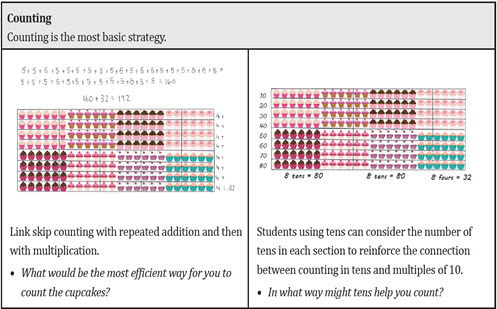Go to website

# reSolve: Multiplication: reSolve Bakery

This series of three lessons explores strategies for multi-digit multiplication. Students are presented with a range of problems in the context of a bakery producing arrays of cupcakes. The lessons aim to develop a range of strategies based on the associative and distributive properties of multiplication, moving students from using an array with all items perceived, to a grid array, and on to an open array and area model for multiplication. Each lesson is outlined in detail including curriculum links, vocabulary, supporting PowerPoint presentations, lists of materials needed, sample answers, discussion points and student resources. This sequence is part of the reSolve: Mathematics by Inquiry program.

Year level(s) Year 5
Audience Teacher
Purpose Teaching resource
Teaching strategies and pedagogical approaches Mathematics investigation
Keywords arrays, multiplication

## Curriculum alignment

Curriculum connections Numeracy
Strand and focus Number, Build understanding, Apply understanding
Topics Multiplication and division
AC: Mathematics (V9.0) content descriptions
AC9M5N06
Solve problems involving multiplication of larger numbers by one- or two-digit numbers, choosing efficient calculation strategies and using digital tools where appropriate; check the reasonableness of answers

Numeracy progression Multiplicative strategies (P7)